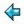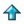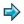### unify.m

Package for doing first order unification, parameterised on an abstract theory of expressions (DT).

see the file polish.m for an example of the use of this package.

```%free { expr,operator,var::type;
isvar::expr->bool; getvar::expr->var; putvar::var->expr;
rator::expr->operator; rands::expr->[expr];
construct::operator->[expr]->expr;
}

||Our theory of expressions is as follows - an expression  is  either  a
||variable,  or  else  it  consists  of  a rator together with a list of
||rands.  So for example a constant will be viewed as a rator which  has
||an  empty  list of rands.  The nature of variables, and the collection
||of possible rators and their arities, is determined at %include  time.
||This enables us to use the same code  for  performing  unification  in
||quite different object languages.

||for each e::expr, one of the following propositions will be true
||either    isvar e & e = putvar(getvar e)
||or       ~isvar e & e = construct(rator e)(rands e)

%export unifyexprs unify ult q

q * ::= FAIL | SUCCEED *  ||useful type operator

unifyexprs :: expr->expr->q expr  ||convenient for testing

unify :: subst->expr->expr->q subst

||this implements the unification algorithm  -  it  takes  a  substition
||(mapping from variables to expressions) and a pair of expressions, and
||returns the least  extension  of  the  substitution  under  which  the
||expressions become the same - or FAIL if there is none.

ult :: subst->expr->expr

||computes the result of applying a substitution to an expression.

unifyexprs x y = f(unify [] x y)
where
f FAIL = FAIL
f (SUCCEED s) = SUCCEED (ult s x)
||note that (ult s y) = (ult s y)
||if the unification succeeds

subst == [(var,expr)]

||We represent a substitution as a list  of  variable-expression  pairs.
||The  representation  is  lazy,  in  the sense that the expressions may
||contain occurrences of the variables in the domain of the substitution
||- this is taken care of in the definition of ult.

unify s x y
= unifier s (ult s x) (ult s y)
where
unifier s x y = SUCCEED s, if x=y
= SUCCEED((getvar x,y):s), if isvar x & ~occurs x y
= SUCCEED((getvar y,x):s), if isvar y & ~occurs y x
= unifylist (SUCCEED s) (rands x) (rands y),
if ~isvar x & ~isvar y & conforms x y
= FAIL, otherwise
unifylist qs [] [] = qs
unifylist (SUCCEED s) (a:x) (b:y) = unifylist (unify s a b) x y
unifylist FAIL x y = FAIL

ult s x = lookup s (getvar x), if isvar x
= construct(rator x)(map (ult s) (rands x)), otherwise
where
lookup [] a = putvar a
lookup ((a,y):t) a = ult s y  ||note recursive call of ult
lookup ((b,y):t) a = lookup t a

occurs y x        || does subexpression y occur in formula x ?
= x=y, if isvar x
= or (map (occurs y) (rands x)), otherwise

conforms x y = rator x = rator y & #rands x = #rands y
```